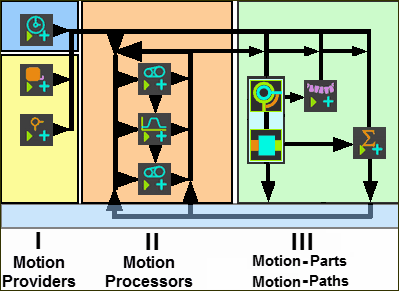﻿ MechDesigner Reference & User Interface > 2.2 Mechanism-Editor: > Kinematic Function-Blocks > Function-Blocks | Connect, Parameter-Values, & Channels

# Function-Blocks | How to connect, their Data-Types & Channels

### Function-Blocks: Wires and Connectors

Function-Blocks have connectors: input-connectors and/or output-connectors. You connect wires from an output-connector of a Function-Block to an input-connector of a different Function-Block.

The motion-data that flows through the wires and Kinematic Function-Blocks has three Data-Channels, The Data-Channels are Position, Velocity and Acceleration, with Linear or Angular motion-units.Schematic: Function-Block Wires and Connectors

The image above is a schematic of how you can add wires to connect Function-Blocks.

### VIDEO : How to Connect Function-Blocks:Video: How to Connect Funtion-Blocks

Data-Types of Motion Function-Blocks.

These Function-Blocks provide or process motion-data.

 Linear-Motion FB Output Data-Type = Rotary Gearing FB Output Data-Type = Input Data-Type Motion FB Output Data-Type = Rotary or Linear Motion-Dimension FB Output Data-Type = Input Data-Type Motion-Path FB No output-connector Input Data-Type = Rotary or Linear Measurement FB Output Data-Type = Rotary or Linear Point-Data FB Output Data-Type = Rotary or Linear

#### Function-Blocks - their Data-Types and Data-Channels

Output-Connector from

Data Type

Data-Channel 1

Data-Channel 2

Data-Channel 3

Linear Motion FB

Rotary

Machine Angle

Machine Angular Velocity

Machine Angular Acceleration

Gearing FB

Linear

Position - mm

Linear Velocity - mm/s

Linear Acceleration - mm/s/s

Rotary

Angle - deg

Angular Velocity deg/s

Angular Acceleration deg/s/s

Motion FB

Linear

Position - mm

Linear Velocity - mm/s

Linear Acceleration - mm/s/s

Rotary

Angle - deg

Angular Acceleration - deg/s/s

Motion-Dimension FB

Linear

Position (mm)

Linear Velocity -mm/s

Linear Acceleration - mm/s/s

Rotary

Angle -deg

Measurement FB

Linear

Position - mm

Linear Velocity - mm/s

Linear Acceleration - mm/s/s

Rotary

Angle -deg

Point FB

Linear

Linear

Linear

X Position mm

Y Position - mm

Magnitude - mm

X Velocity - mm/s

Y Velocity - mm/s

Mag. Velocity- mm/s

X Acceleration - mm/s/s

Y Acceleration - mm/s/s

Mag. Acceleration - mm/s/s

Cam-Data FB

Rotary

Pressure Angle - deg

Contact Pressure Angel 1 - deg

Contact Pressure Angle 2 - deg

Linear

------

Force

Contact Force Total - N

Contact Force, X component - N

Contact Force, Y component

Stress/Pressure

Inner Cam Shear Stress- N/mm2

Outer Cam Shear Stress - N/mm2

------

Linear

Sliding-Velocity (mm/s)

------

------

Force-Data FB

Force

Joint Total Force / Torque

X Component - N

Y Component - N

Force

Cam Contact Force - N

X Component - N

Y Component  - N

Force

Spring Force - N

X Component - N

Y Component - N

Math FB